# SAT II Math II : Absolute Value

## Example Questions

1 3 Next →

### Example Question #84 : Sat Subject Test In Math Ii

Solve: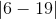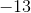Explanation:

The lines on the outside of this problem indicate it is an absolute value problem. When solving with absolute value, remember that it is a measure of displacement from 0, meaning the answer will always be positive.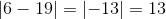For this problem, that gives us a final answer of 13.

### Example Question #85 : Sat Subject Test In Math Ii

Solve: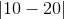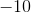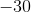Explanation:

The lines on the outside of this problem indicate it is an absolute value problem. When solving with absolute value, remember that it is a measure of displacement from 0, meaning the answer will always be positive.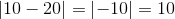For this problem, that gives us a final answer of 10.

### Example Question #86 : Sat Subject Test In Math Ii

Solve: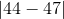Explanation:

The lines on the outside of this problem indicate it is an absolute value problem. When solving with absolute value, remember that it is a measure of displacement from 0, meaning the answer will always be positive.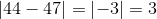For this problem, that gives us a final answer of 3.

### Example Question #87 : Sat Subject Test In Math Ii

Solve: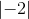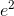Explanation:

The lines on the outside of this problem indicate it is an absolute value problem. When solving with absolute value, remember that it is a measure of displacement from 0, meaning the answer will always be positive.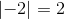For this problem, that gives us a final answer of 2.

### Example Question #81 : Sat Subject Test In Math Ii

Solve: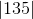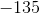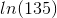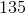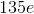Explanation:

The lines on the outside of this problem indicate it is an absolute value problem. When solving with absolute value, remember that it is a measure of displacement from 0, meaning the answer will always be positive.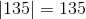For this problem, that gives us a final answer of 135.

### Example Question #21 : Absolute Value

Solve: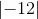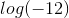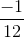Explanation:

The lines on the outside of this problem indicate it is an absolute value problem. When solving with absolute value, remember that it is a measure of displacement from 0, meaning the answer will always be positive.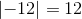For this problem, that gives us a final answer of 12.

### Example Question #21 : Absolute Value

Solve: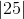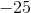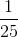Explanation:

The lines on the outside of this problem indicate it is an absolute value problem. When solving with absolute value, remember that it is a measure of displacement from 0, meaning the answer will always be positive.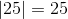For this problem, that gives us a final answer of 25.

1 3 Next →

### All SAT II Math II Resources